The COUNTREG Procedure

SCORE Statement

(Experimental)

• SCORE <OUT=SAS-data-set> <output-options>;

The SCORE statement enables you to compute predicted values and other statistics for a SAS data set. As with the OUTPUT statement, the new data set that is created contains all the variables in the input data set and, optionally, the estimates of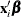, the expected value of the response variable, and the probability that the response variable will take the current value or other values that you specify. In a zero-inflated model, you can additionally request that the output data set contain the estimates of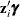and the probability that the response is zero as a result of the zero-generating process. For the Conway-Maxwell-Poisson model, the estimates of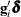,,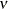,, mode, variance, and dispersion are also available. Except for the probability of the current value, these statistics can be computed for all observations in which the regressors are not missing, even if the response is missing.

The following statements fit a Poisson model by using the DocVisit data set. Additional observations in the additionalPatients data set are used to compute expected values by using the SCORE statement. The data in the additionalPatients data set are not used during the fitting stage and are used only for scoring.

You score a data set in two separate steps. In the first step, you fit the model and use the STORE statement to preserve it in the DocVisitPoisson item store, as shown in the following statements:

   proc countreg data=docvisit;
model doctorvisits=sex illness income / dist=poisson;
store docvisitPoisson;
run;


In the second step, you retrieve the content of the DocVisitPoisson item store and use it to calculate expected values by using the SCORE statement for the additionalPatients data set as follows:

   proc countreg restore=docvisitPoisson data=additionalPatients;
score out=outScores mean=meanPoisson probability=prob;
run;


By retrieving the model from the item store and using it in a postprocessing step, you can separate the fitting and scoring stages and use data for scoring that might not be available at the time when the model was fitted.

You can specify only one SCORE statement. You can specify the following output-options:

OUT=SAS-data-set

names the output data set.

XBETA=name

names the variable that contains estimates of.

PRED=name
MEAN=name

assigns a name to the variable that contains the predicted value of the response variable.

PROB=name

names the variable that contains the probability that the response variable will take the current value, Pr(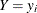).

PROBCOUNT(value1 <value2...>)

outputs the probability that the response variable will take particular values. Each value should be a nonnegative integer. Nonintegers are rounded to the nearest integer. The value can also be a list of the form X TO Y BY Z. For example, PROBCOUNT(0 1 2 TO 10 BY 2 15) requests predicted probabilities for counts 0, 1, 2, 4, 5, 6, 8, 10, and 15. This option is not available for the fixed-effects and random-effects panel models.

ZGAMMA=name

names the variable that contains estimates of.

PROBZERO=name

names the variable that contains the value of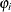, the probability of the response variable taking on the value of zero as a result of the zero-generating process. It is written to the output file only if the model is zero-inflated. This is not the overall probability of a zero response; that is provided by the PROBCOUNT(0) option.

GDELTA=name

assigns a name to the variable that contains estimates offor the Conway-Maxwell-Poisson distribution.

LAMBDA=name

assigns a name to the variable that contains the estimate offor the Conway-Maxwell-Poisson distribution.

NU=name

assigns a name to the variable that contains the estimate offor the Conway-Maxwell-Poisson distribution.

MU=name

assigns a name to the variable that contains the estimate offor the Conway-Maxwell-Poisson distribution.

MODE=name

assigns a name to the variable that contains the integral part of(mode) for the Conway-Maxwell-Poisson distribution.

VARIANCE=name

assigns a name to the variable that contains the estimate of variance for the Conway-Maxwell-Poisson distribution.

DISPERSION=name

assigns a name to the variable that contains the value of dispersion for the Conway-Maxwell-Poisson distribution.